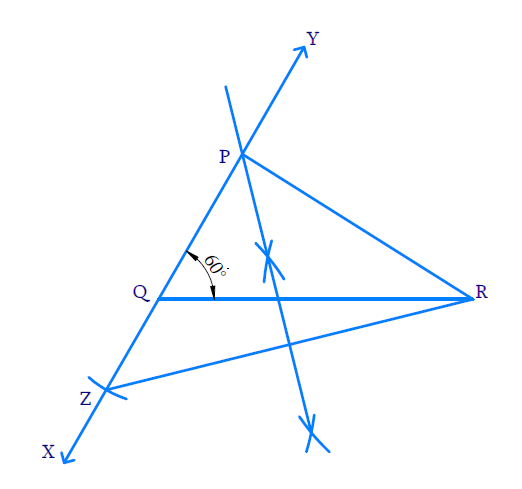# Ex.11.2 Q3 Constructions Solution - NCERT Maths Class 9

## Question

Construct a triangle $$PQR$$ in which $$QR=6\;\mathrm{cm}, Q=\angle 60^{\circ}$$ and $$PR-PQ= 2\;\rm{cm}.$$

Video Solution
Constructions
Ex 11.2 | Question 3

## Text Solution

Steps of Construction:(i) Draw line  $$QR =6\;\rm{cm}$$ . Make an angle of $$60^{\circ}$$ at a point $$Q$$ using a protractor and extend it below line $$QR$$.

(ii) With $$Q$$ as center and radius as $$2\;\rm{cm}$$, draw an arc to intersect the ray $$QX$$ at $$Z$$

(iii) Join $$RZ$$

(iv) With $$Z$$ and $$R$$ as centres and radius greater than half of $$ZR$$, draw arcs on either side to intersect each other.

(v) Join the intersecting points and extend it to meet the ray $$QY$$ at $$P$$.

(vi) Join $$P$$ and $$R$$ .$$PQR$$ is the required triangle.

Learn from the best math teachers and top your exams

• Live one on one classroom and doubt clearing
• Practice worksheets in and after class for conceptual clarity
• Personalized curriculum to keep up with school